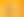Keeping it in Proportion: Fractions and Decimals

# Stages1. Fractions as Equal Parts of a Whole

EXPLORE

Suggested Learning Intentions

• To understand that fractions can represent equal parts of a whole

Sample Success Criteria

• I can accurately identify the name of each fraction part
• I can correctly name fractions using the number of equal parts needed to make up the whole
• I can explain and justify my solutions using a variety of manipulatives
EXPLORE

2. Chocolate Cake

EXPLORE

Suggested Learning Intentions

• To understand fractions result from division
• To compare fractions, noting how the numerator and denominator affect the size of a fraction

Sample Success Criteria

• I can identify fractions that have different names but are the same size
• I can identify which fraction is larger or smaller based on the name of the parts and how many of these parts there are
• I can justify my thinking and solutions using manipulatives or other representations
EXPLORE

3. Fractions to Decimals

EXPLORE

Suggested Learning Intentions

• To estimate and locate fractions and decimals on a number line
• To understand fractions and decimals as different representations of the same number

Sample Success Criteria

• I can locate simple fractions, improper fractions, and decimals on a number line
• I can represent fractions as decimals using different models: area models, number lines and set models
• I can demonstrate my understanding using a variety of manipulatives
EXPLORE

4. Colour in Fractions

EXPLORE

Suggested Learning Intentions

• To understand two fractions can be the same size but have different names

Sample Success Criteria

• I can identify fractions that are the same size but have different names
• I can make fractions that are equal in size using different parts
• I can use manipulatives to model and explain my thinking and solutions
EXPLORE

5. Colour in Decimats

EXPLORE

Suggested Learning Intentions

• To understand place value in decimals
• To understand how place value determines the size of a decimal

Sample Success Criteria

• I can accurately colour decimals on a decimat to demonstrate place value
• I can order decimals correctly on a number line
• I can use different manipulatives to model and explain my solutions and thinking
EXPLORE